# MSBSHSE Solutions For Class 8 Maths Part 1 Chapter 6- Factorisation of Algebraic Expressions

MSBSHSE Solutions For Class 8 Maths Part 1 Chapter 6 – Factorisation of Algebraic Expressions are provided here to help students come out with flying colours in their board examinations. As students are familiar with numbers, their problem-solving and analytical skills have improved considerably. We all know that algebra plays an essential role in maths. This chapter discusses the concept factors of a quadratic trinomial, rational algebraic expressions. Here in Maharashtra State Board Class 8 Textbooks Part 1 such problems are solved. Our expert faculty team has solved the questions in a step by step format, which helps students understand the concepts clearly. These solutions will also help them in obtaining knowledge and strong command over the subject. If the students wish to secure an excellent score then solving MSBSHSE Solutions Class 8 is a must. Students can refer to and download the PDF of Maharashtra Board Solutions for Class 8 Maths Chapter 6 Factorisation of Algebraic Expressions, which is readily available from the links.

## Download the PDF of Maharashtra Board Solutions For Class 8 Maths Part 1 Chapter 6- Factorisation of Algebraic Expressions.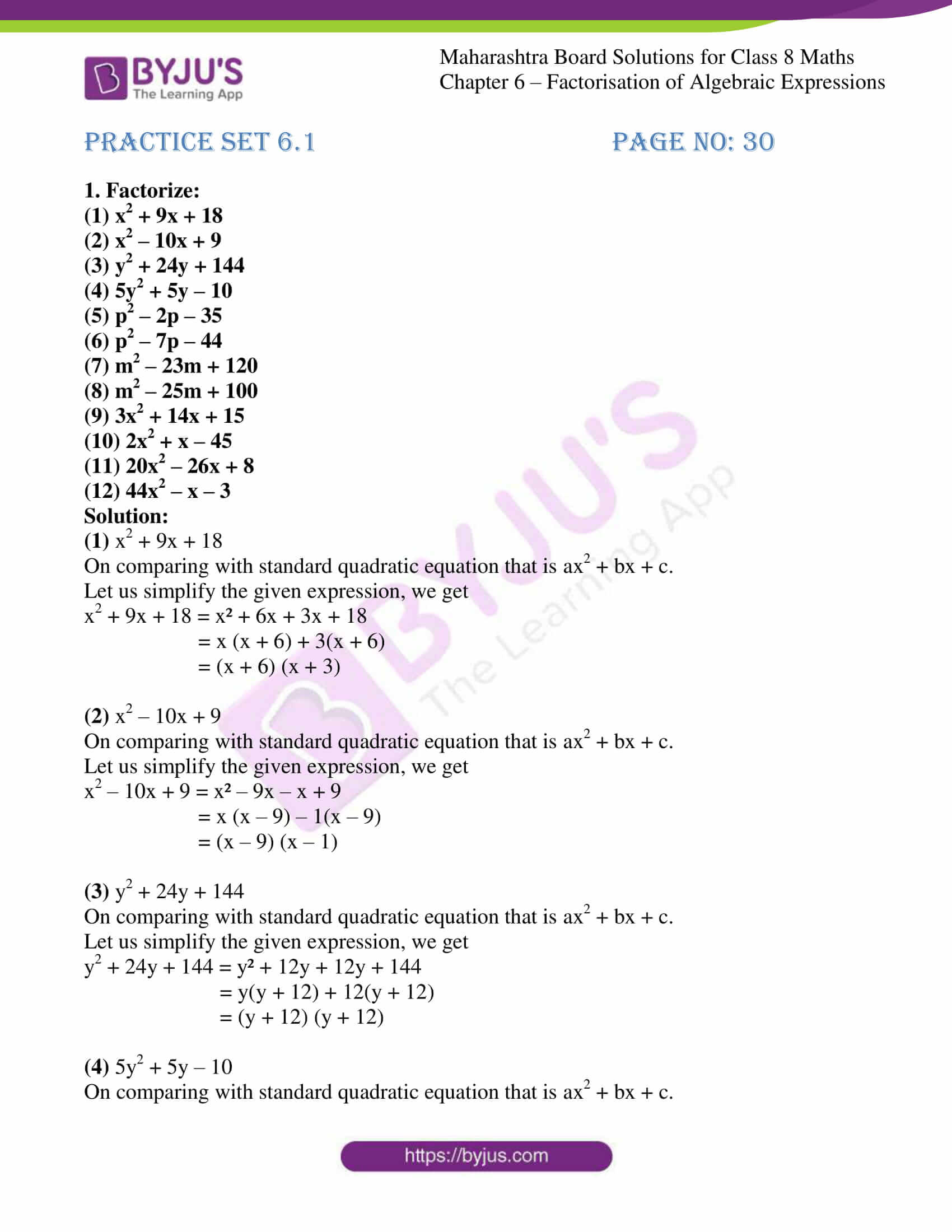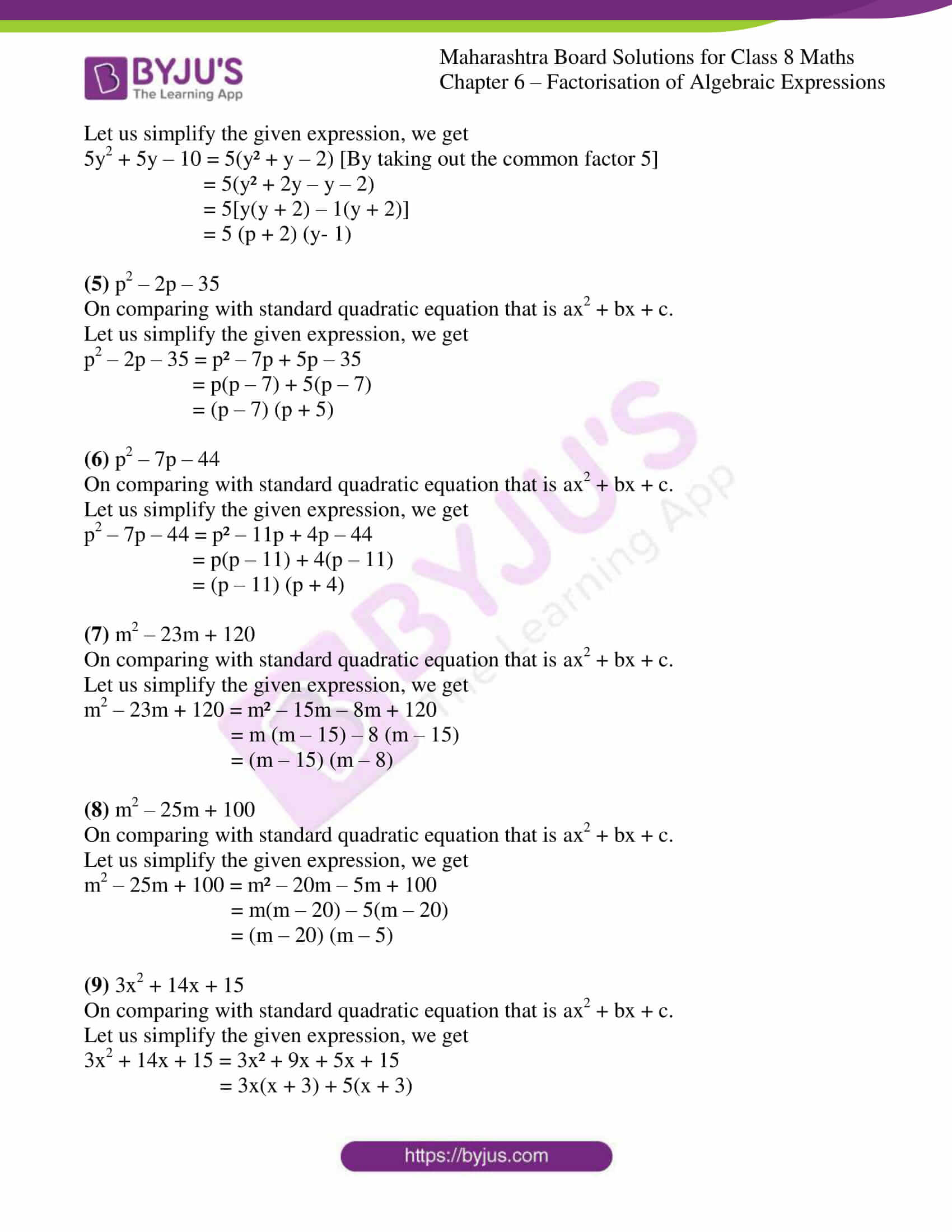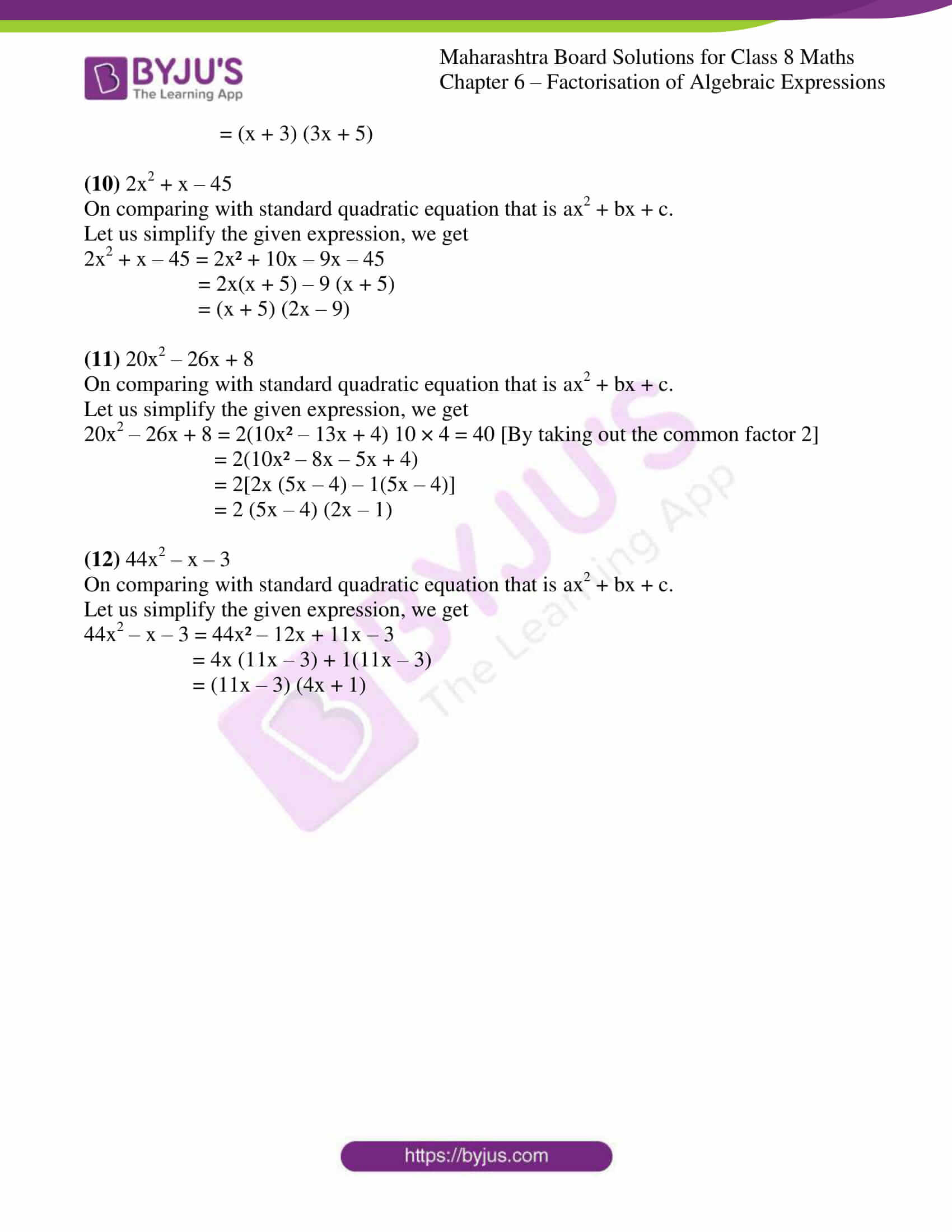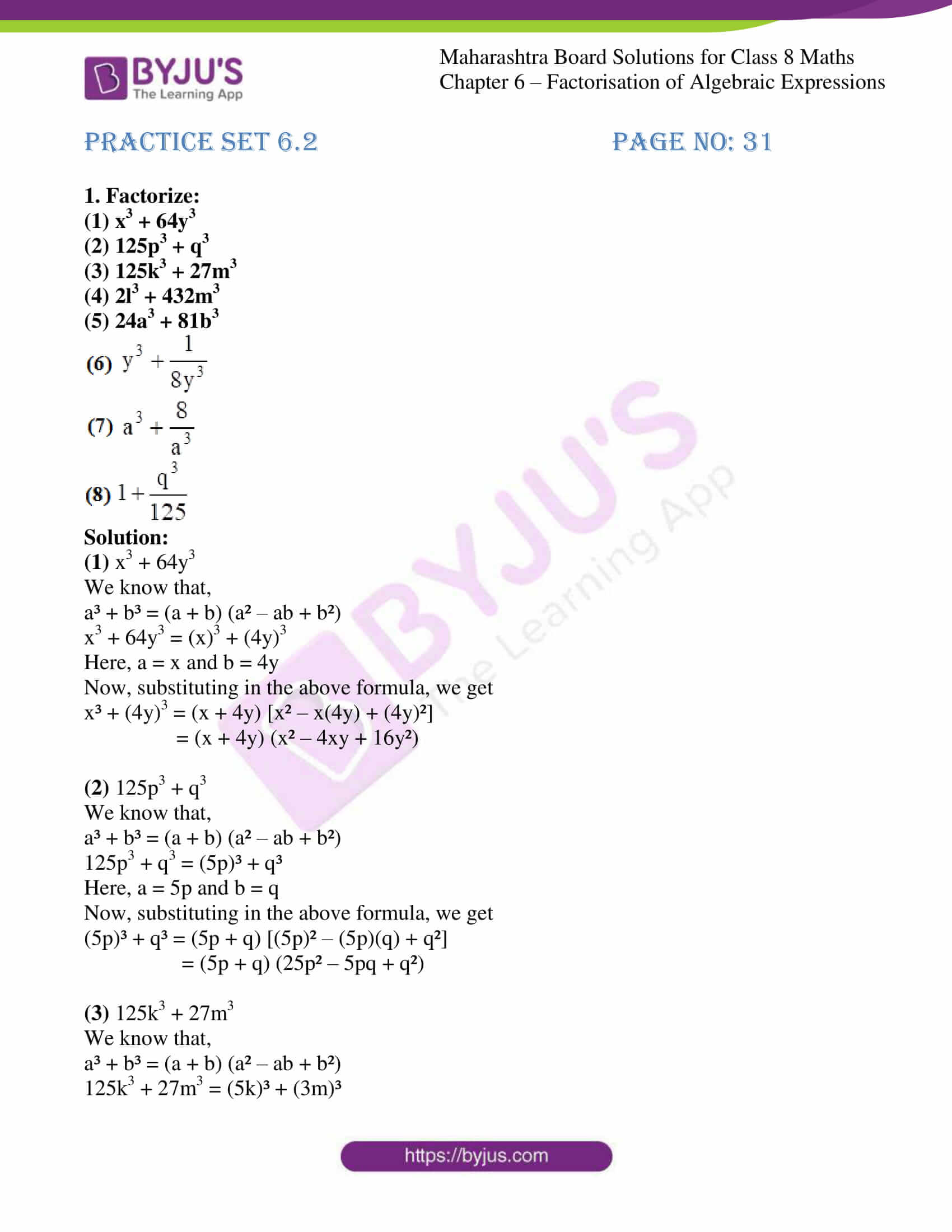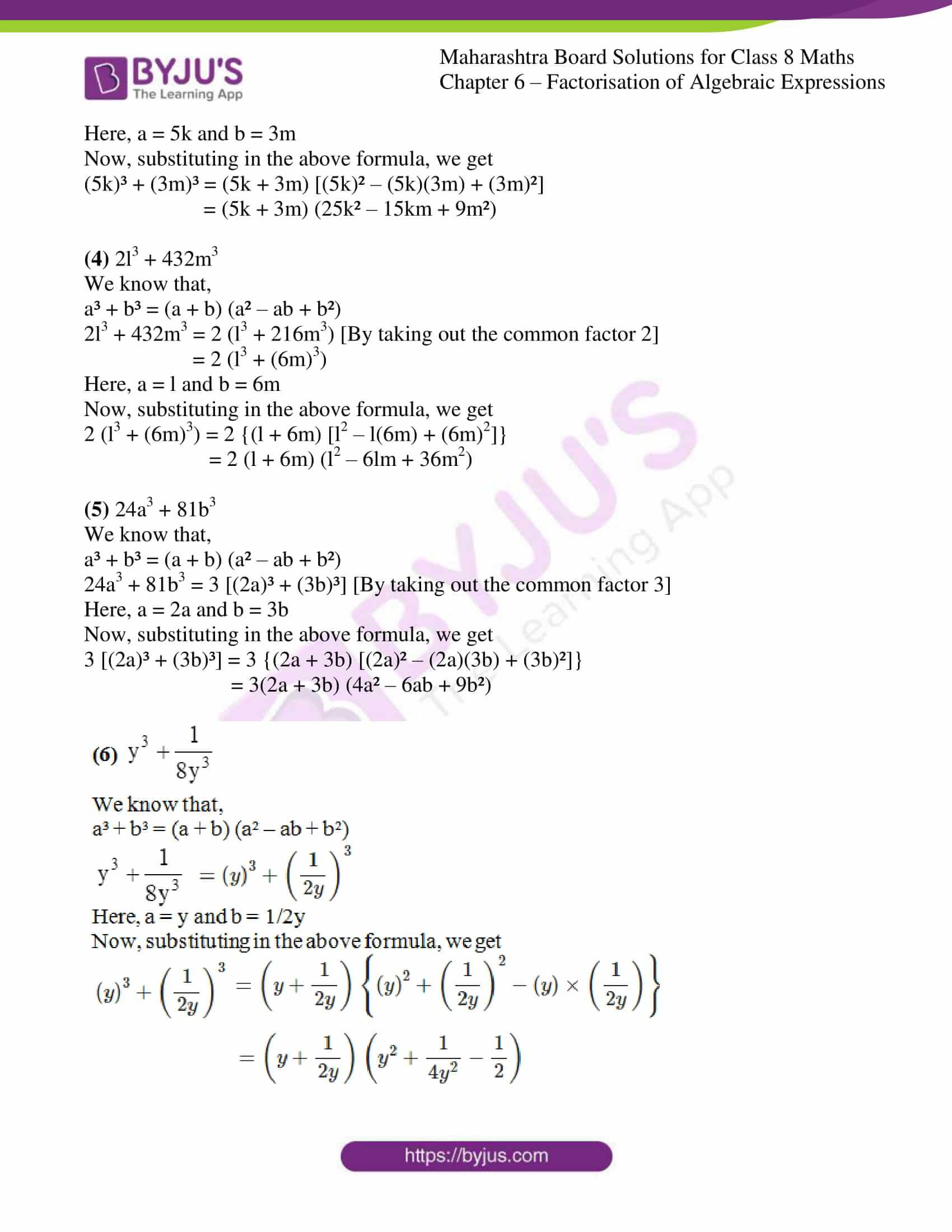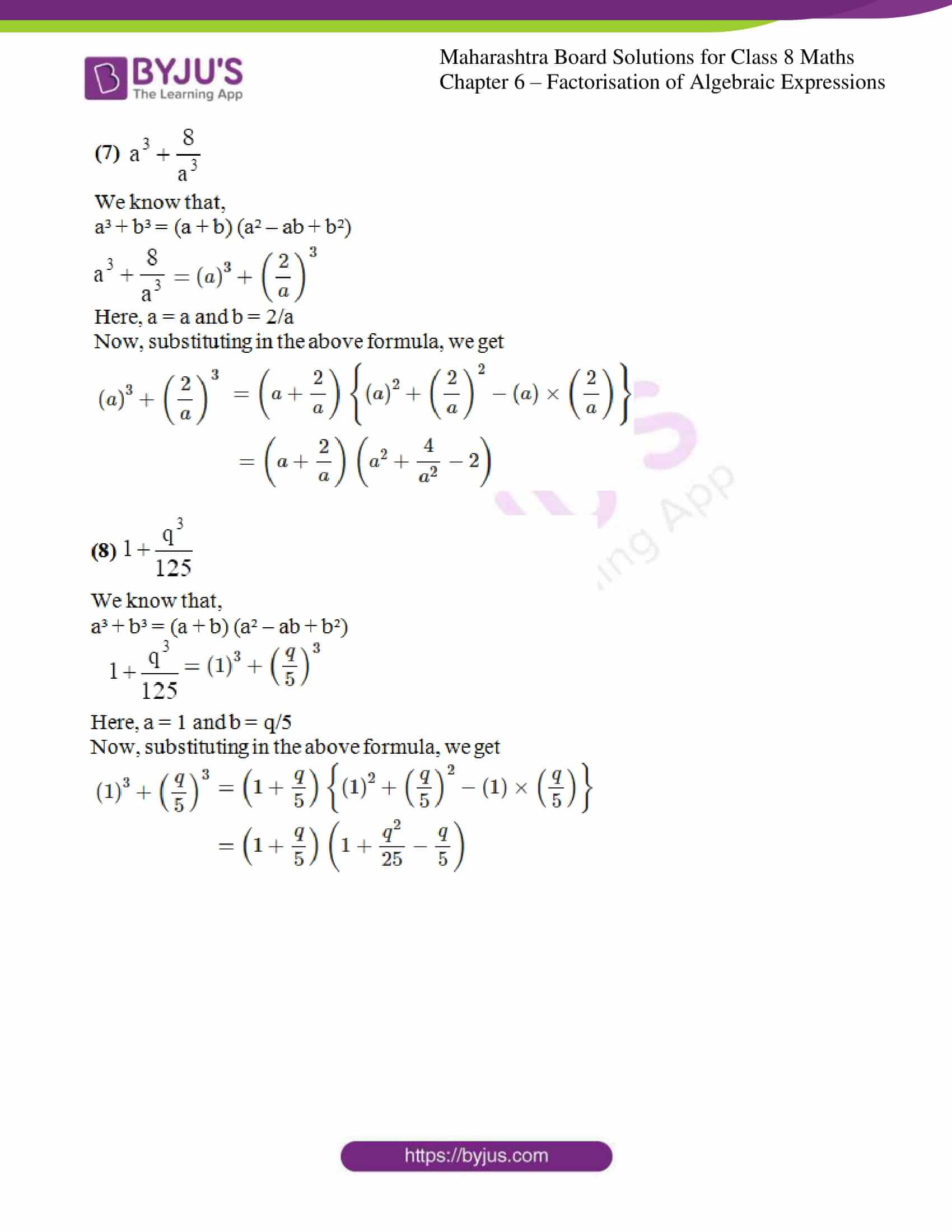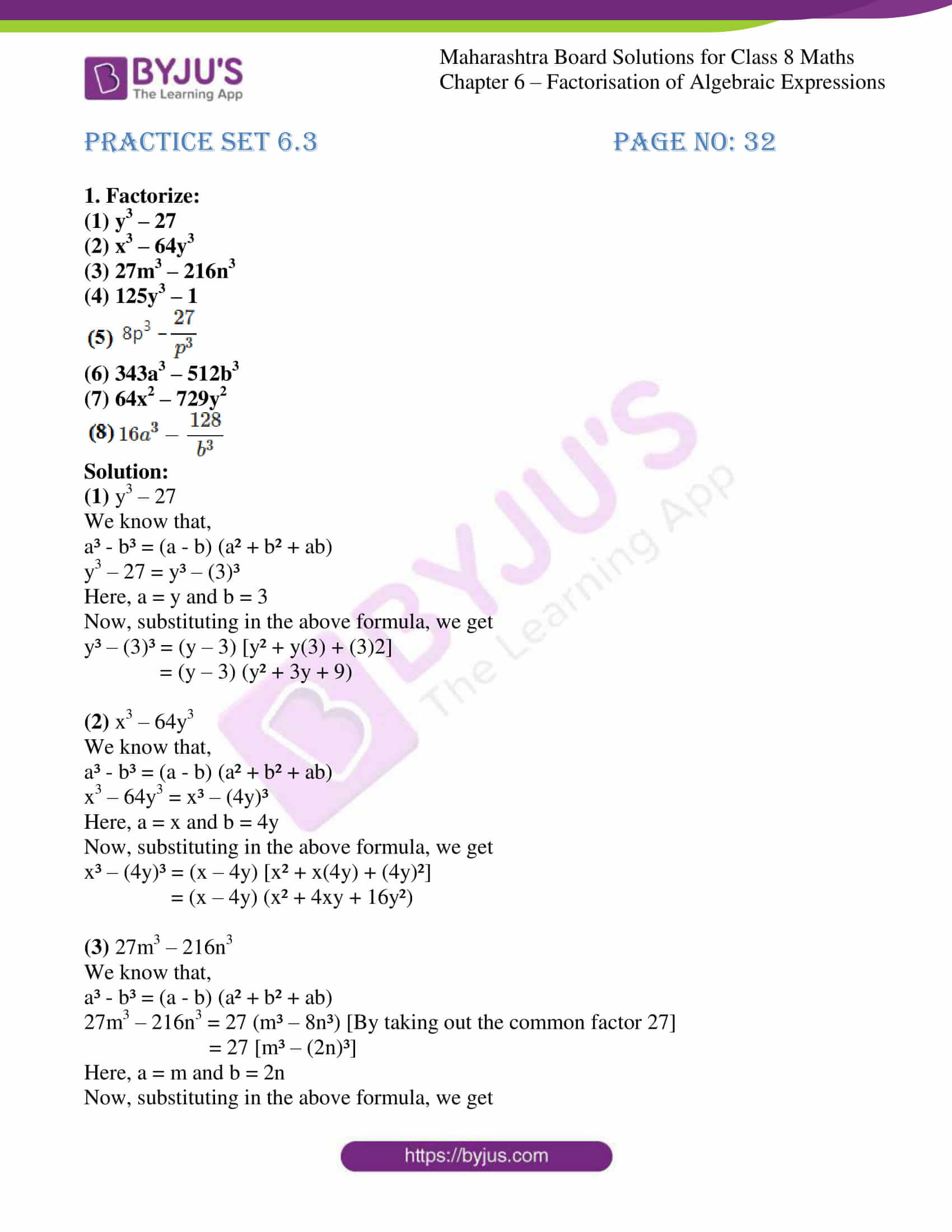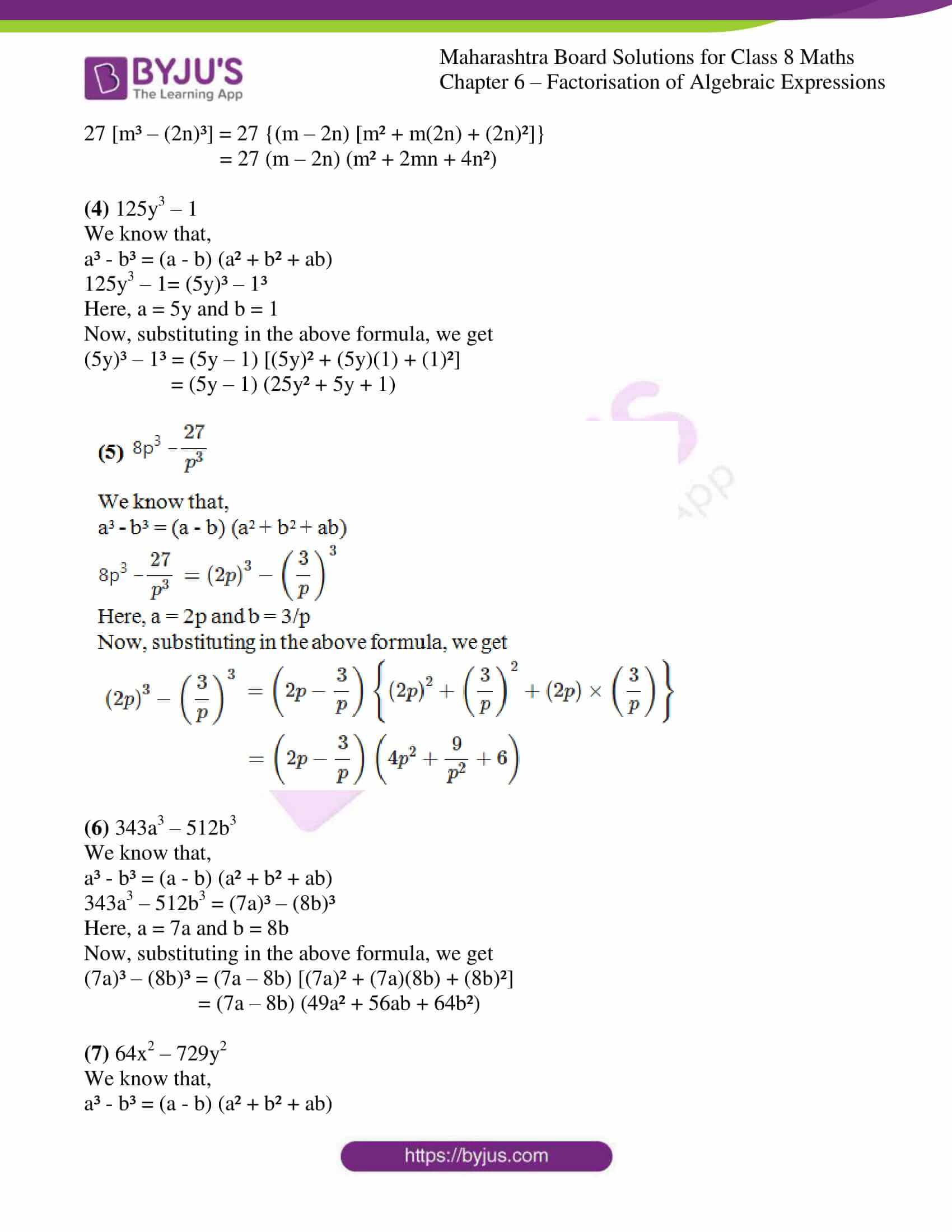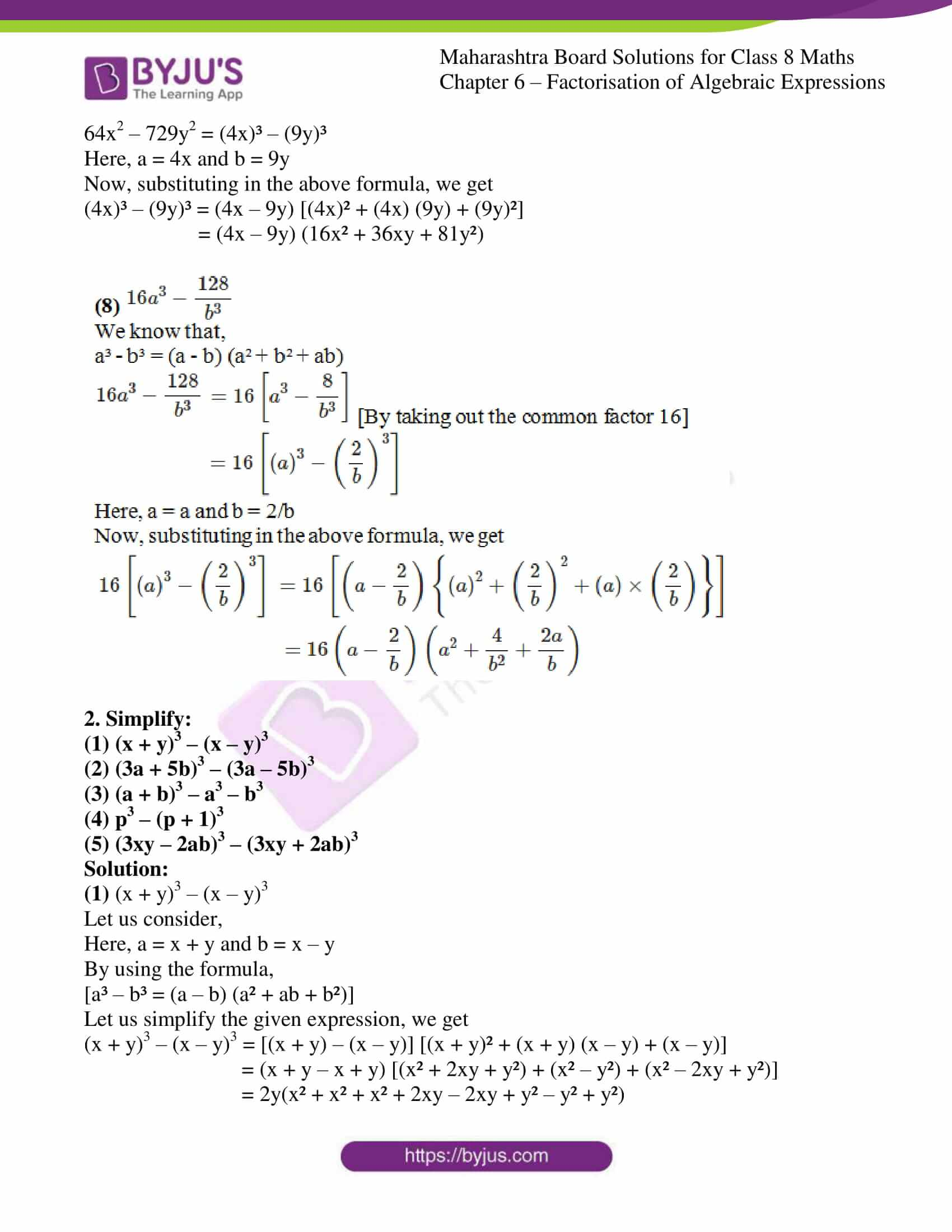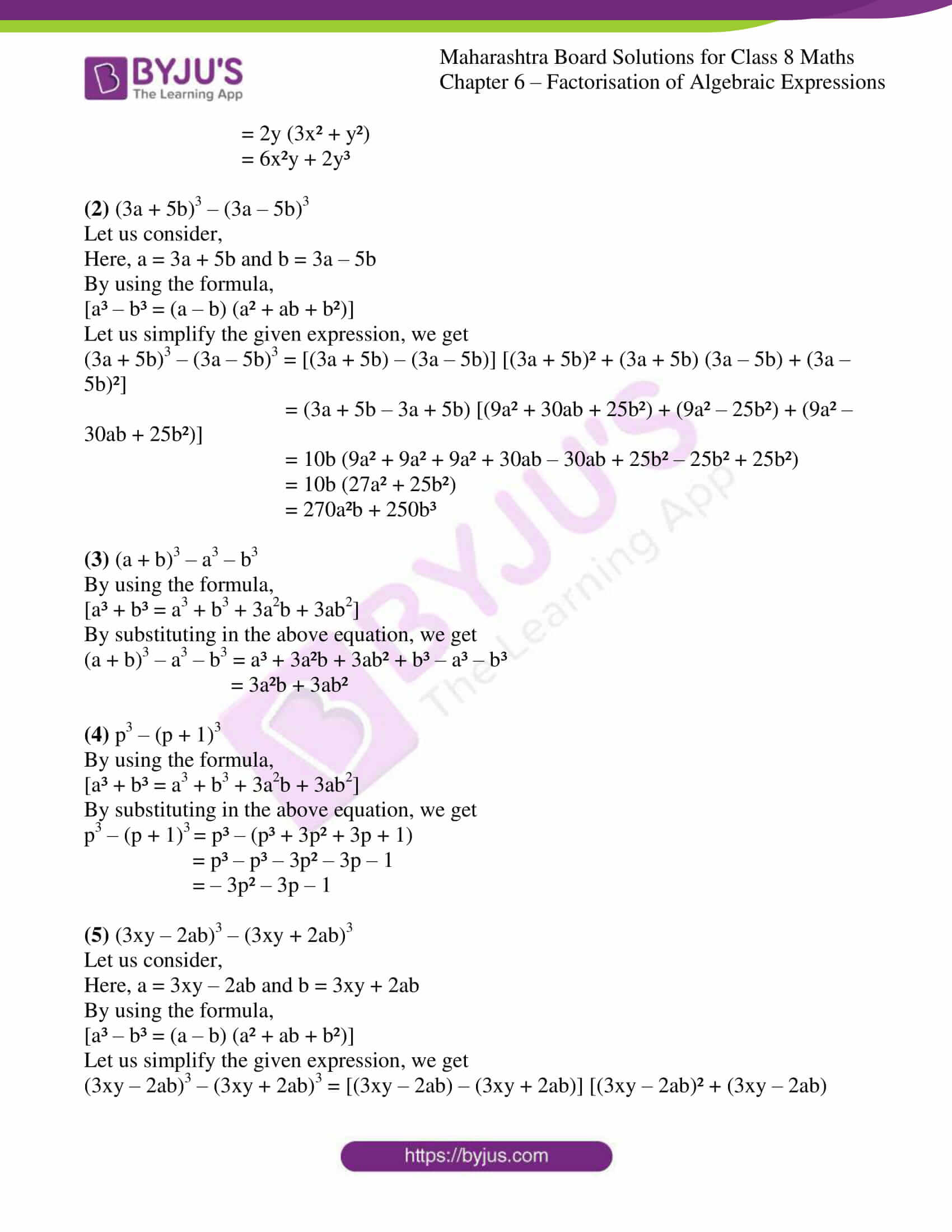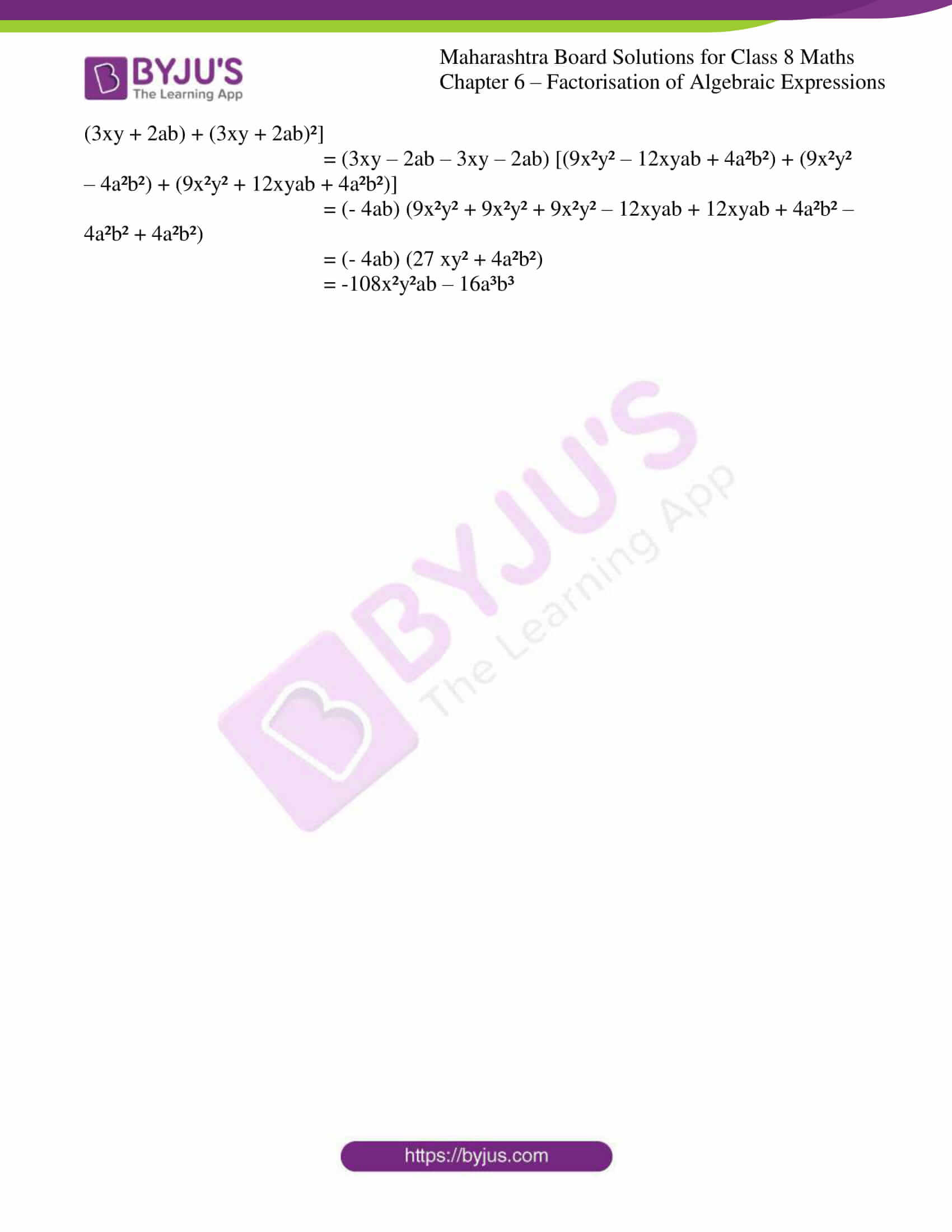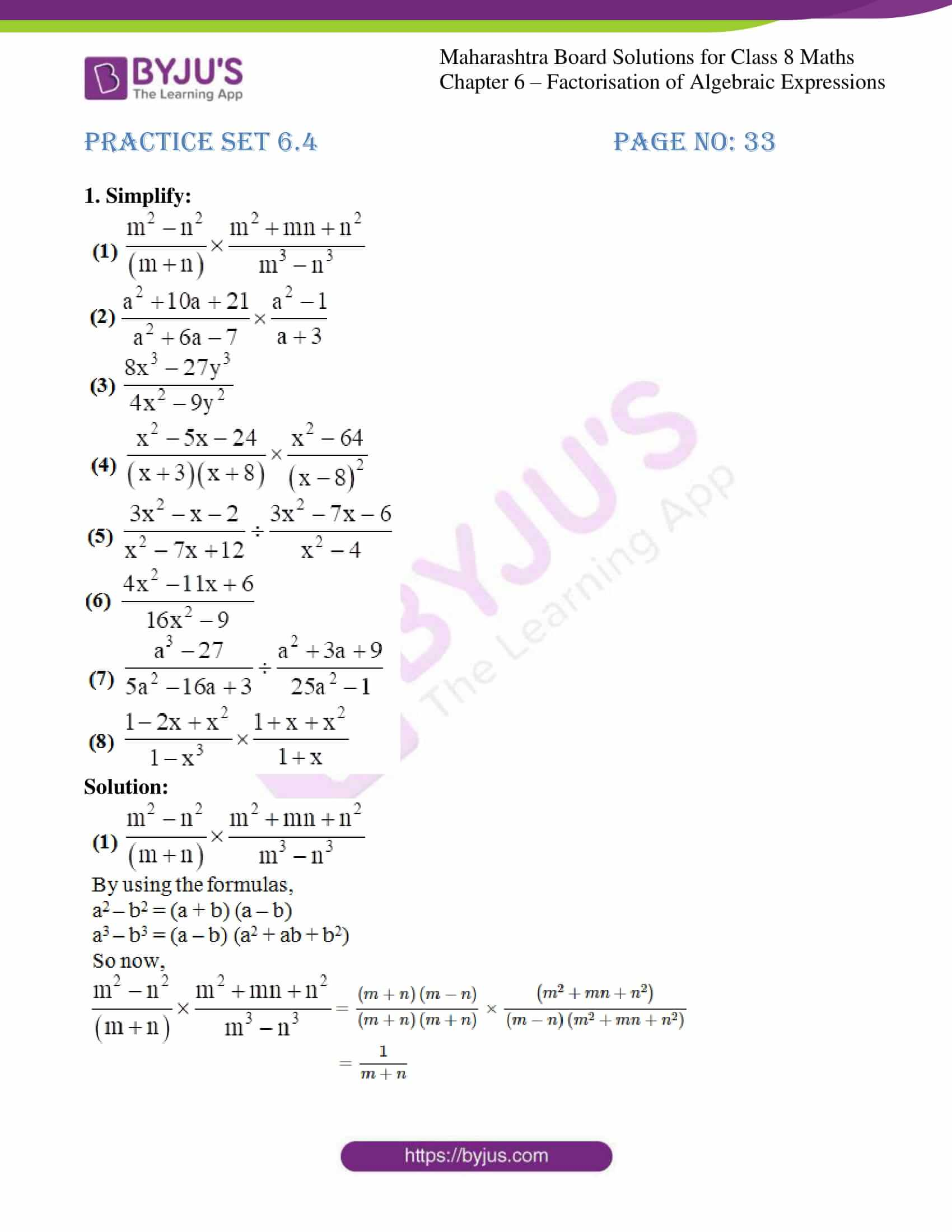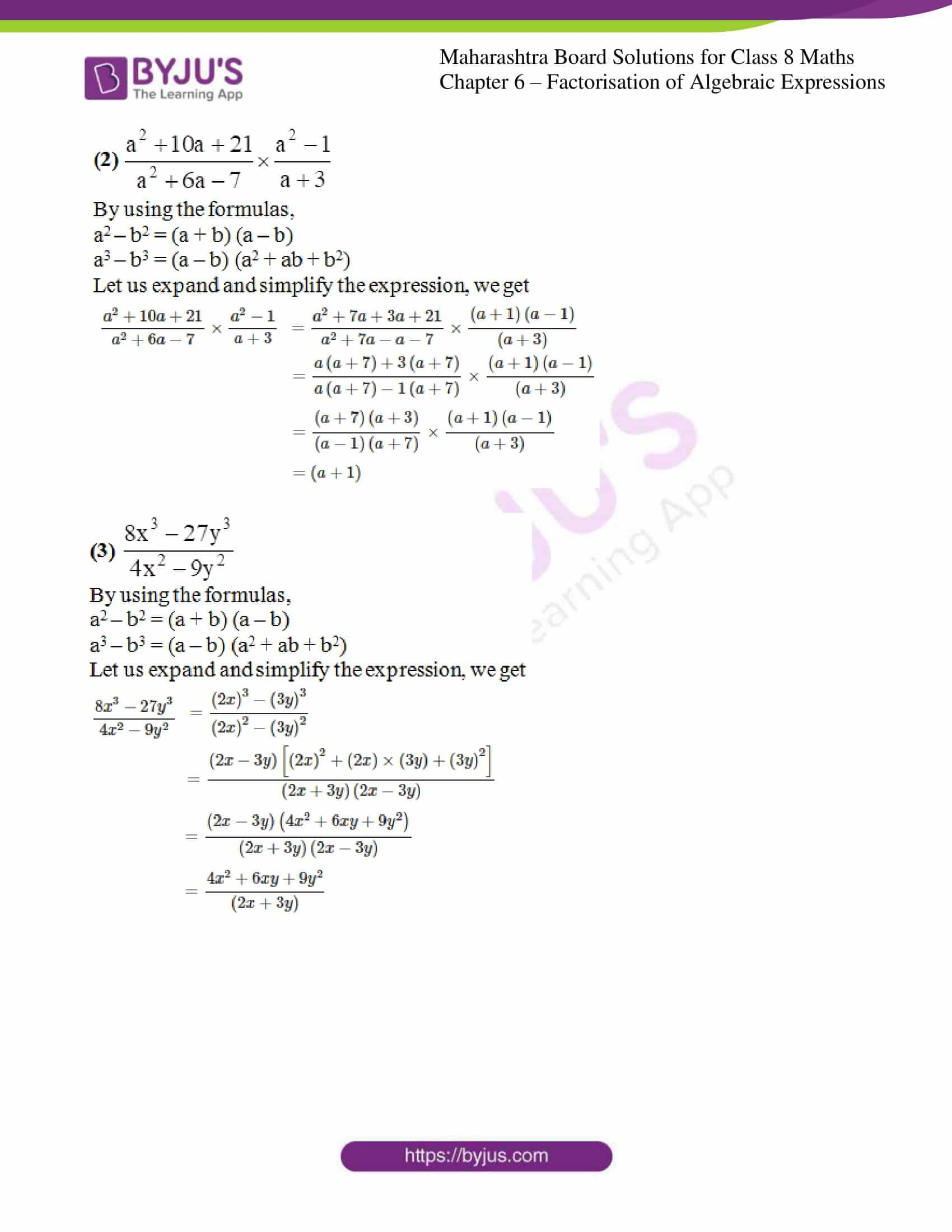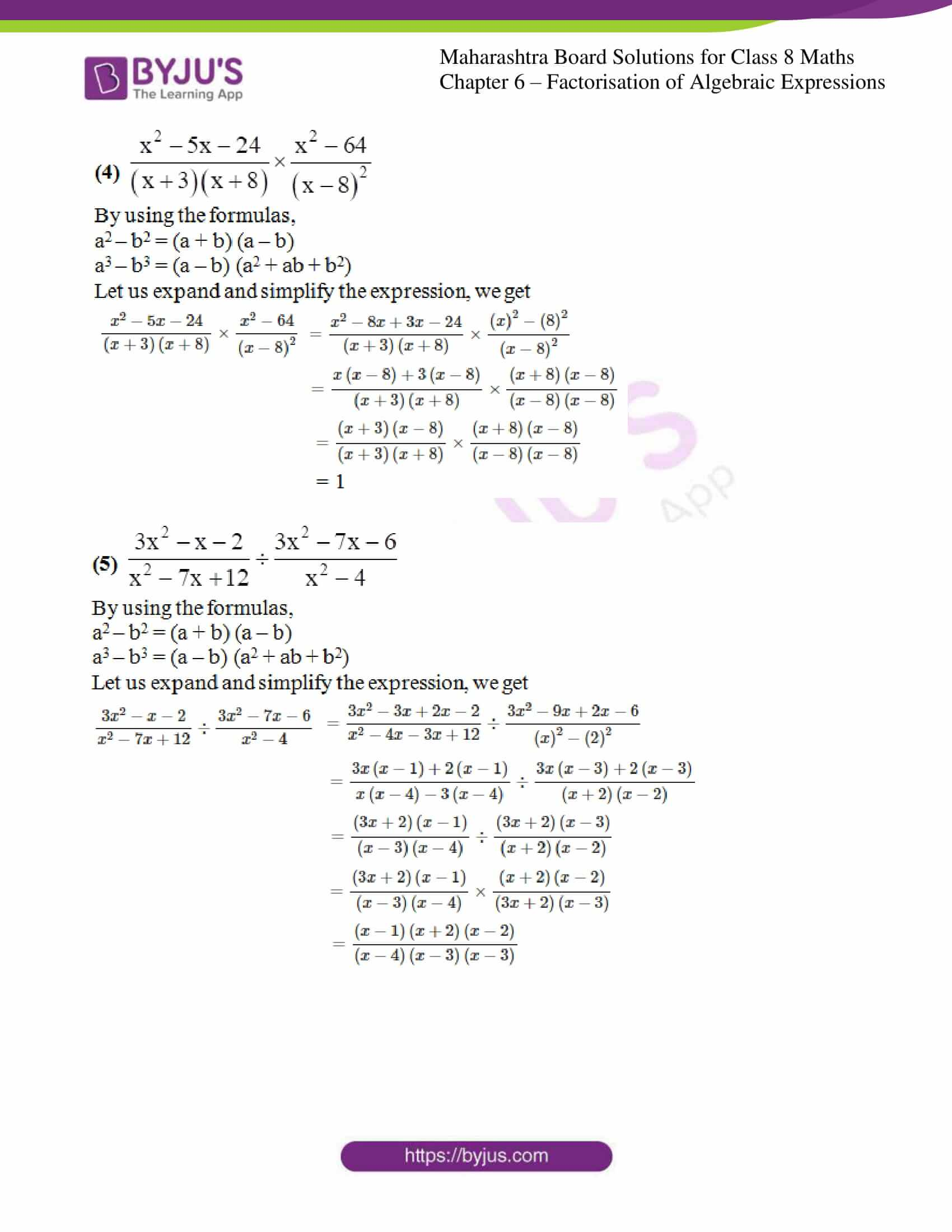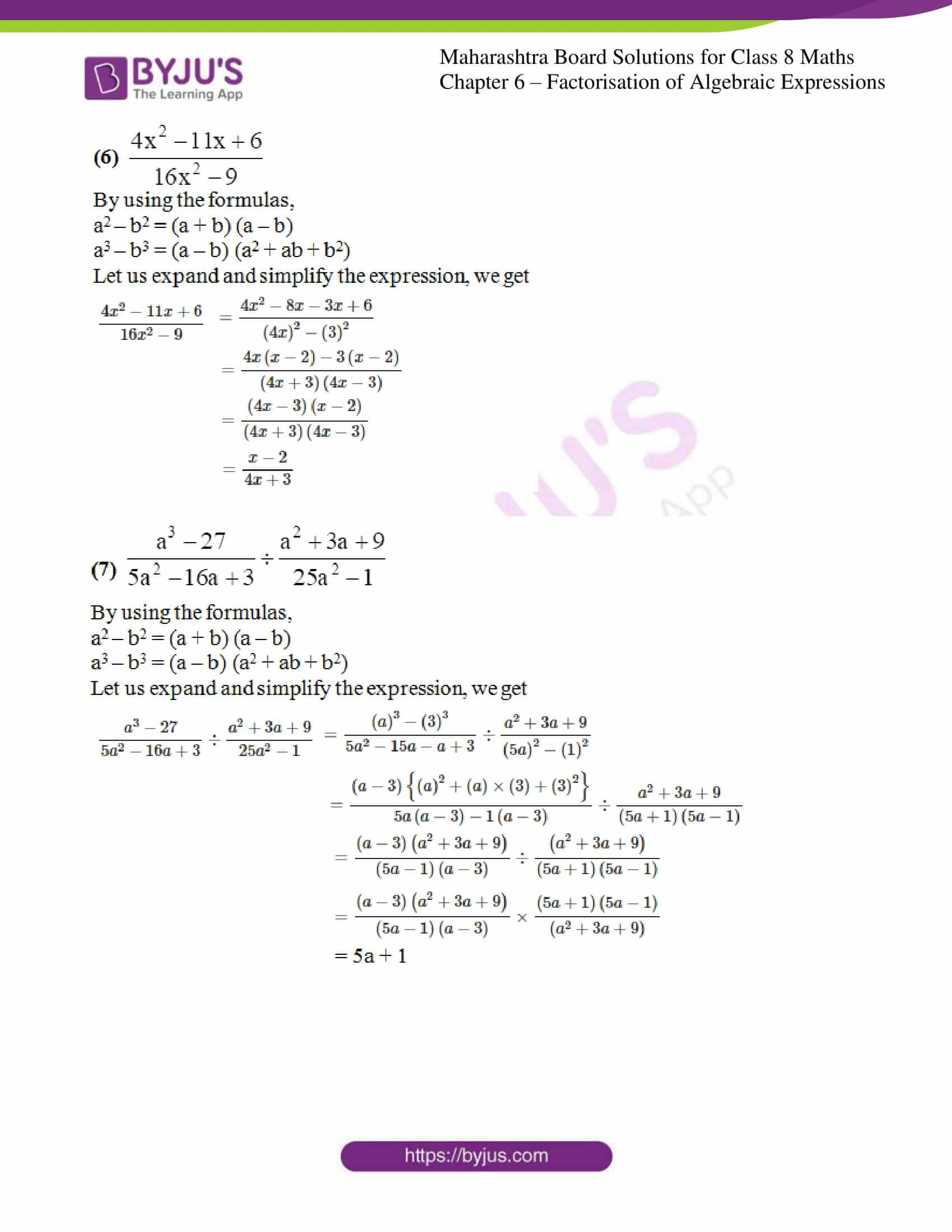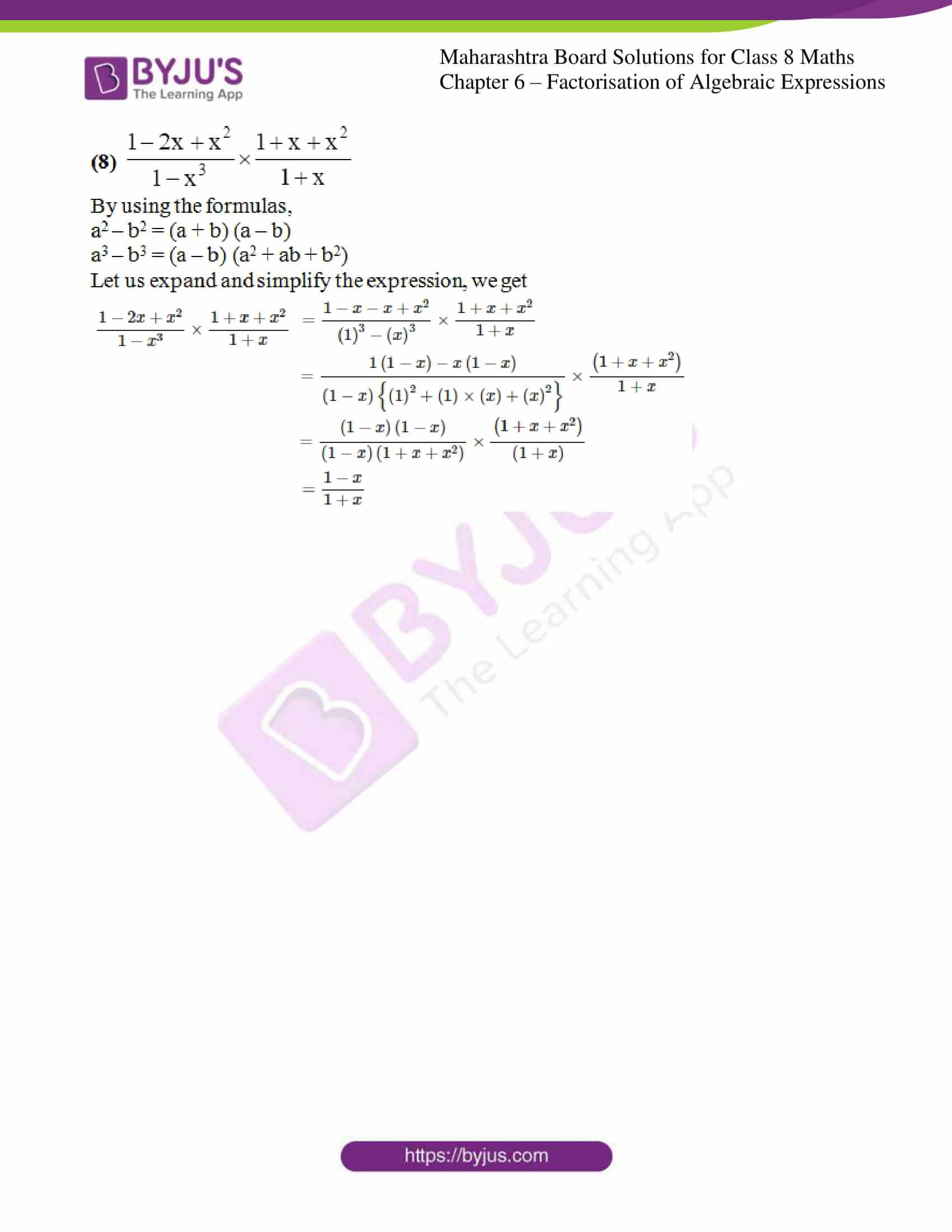### Access answers to Maharashtra Board Solutions For Class 8 Maths Part 1 Chapter 6- Factorisation of Algebraic Expressions.

Practice set 6.1 PAGE NO: 30

1. Factorize:

(1) x2 + 9x + 18

(2) x2 – 10x + 9

(3) y2 + 24y + 144

(4) 5y2 + 5y – 10

(5) p2 – 2p – 35

(6) p2 – 7p – 44

(7) m2 – 23m + 120

(8) m2 – 25m + 100

(9) 3x2 + 14x + 15

(10) 2x2 + x – 45

(11) 20x2 – 26x + 8

(12) 44x2 – x – 3

Solution:

(1) x2 + 9x + 18

On comparing with standard quadratic equation that is ax2 + bx + c.

Let us simplify the given expression, we get

x2 + 9x + 18 = x² + 6x + 3x + 18

= x (x + 6) + 3(x + 6)

= (x + 6) (x + 3)

(2) x2 – 10x + 9

On comparing with standard quadratic equation that is ax2 + bx + c.

Let us simplify the given expression, we get

x2 – 10x + 9 = x² – 9x – x + 9

= x (x – 9) – 1(x – 9)

= (x – 9) (x – 1)

(3) y2 + 24y + 144

On comparing with standard quadratic equation that is ax2 + bx + c.

Let us simplify the given expression, we get

y2 + 24y + 144 = y² + 12y + 12y + 144

= y(y + 12) + 12(y + 12)

= (y + 12) (y + 12)

(4) 5y2 + 5y – 10

On comparing with standard quadratic equation that is ax2 + bx + c.

Let us simplify the given expression, we get

5y2 + 5y – 10 = 5(y² + y – 2) [By taking out the common factor 5]

= 5(y² + 2y – y – 2)

= 5[y(y + 2) – 1(y + 2)]

= 5 (p + 2) (y- 1)

(5) p2 – 2p – 35

On comparing with standard quadratic equation that is ax2 + bx + c.

Let us simplify the given expression, we get

p2 – 2p – 35 = p² – 7p + 5p – 35

= p(p – 7) + 5(p – 7)

= (p – 7) (p + 5)

(6) p2 – 7p – 44

On comparing with standard quadratic equation that is ax2 + bx + c.

Let us simplify the given expression, we get

p2 – 7p – 44 = p² – 11p + 4p – 44

= p(p – 11) + 4(p – 11)

= (p – 11) (p + 4)

(7) m2 – 23m + 120

On comparing with standard quadratic equation that is ax2 + bx + c.

Let us simplify the given expression, we get

m2 – 23m + 120 = m² – 15m – 8m + 120

= m (m – 15) – 8 (m – 15)

= (m – 15) (m – 8)

(8) m2 – 25m + 100

On comparing with standard quadratic equation that is ax2 + bx + c.

Let us simplify the given expression, we get

m2 – 25m + 100 = m² – 20m – 5m + 100

= m(m – 20) – 5(m – 20)

= (m – 20) (m – 5)

(9) 3x2 + 14x + 15

On comparing with standard quadratic equation that is ax2 + bx + c.

Let us simplify the given expression, we get

3x2 + 14x + 15 = 3x² + 9x + 5x + 15

= 3x(x + 3) + 5(x + 3)

= (x + 3) (3x + 5)

(10) 2x2 + x – 45

On comparing with standard quadratic equation that is ax2 + bx + c.

Let us simplify the given expression, we get

2x2 + x – 45 = 2x² + 10x – 9x – 45

= 2x(x + 5) – 9 (x + 5)

= (x + 5) (2x – 9)

(11) 20x2 – 26x + 8

On comparing with standard quadratic equation that is ax2 + bx + c.

Let us simplify the given expression, we get

20x2 – 26x + 8 = 2(10x² – 13x + 4) 10 × 4 = 40 [By taking out the common factor 2]

= 2(10x² – 8x – 5x + 4)

= 2[2x (5x – 4) – 1(5x – 4)]

= 2 (5x – 4) (2x – 1)

(12) 44x2 – x – 3

On comparing with standard quadratic equation that is ax2 + bx + c.

Let us simplify the given expression, we get

44x2 – x – 3 = 44x² – 12x + 11x – 3

= 4x (11x – 3) + 1(11x – 3)

= (11x – 3) (4x + 1)

Practice set 6.2 PAGE NO: 31

1. Factorize:

(1) x3 + 64y3

(2) 125p3 + q3

(3) 125k3 + 27m3

(4) 2l3 + 432m3

(5) 24a3 + 81b3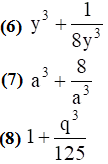Solution:

(1) x3 + 64y3

We know that,

a³ + b³ = (a + b) (a² – ab + b²)

x3 + 64y3 = (x)3 + (4y)3

Here, a = x and b = 4y

Now, substituting in the above formula, we get

x³ + (4y)3 = (x + 4y) [x² – x(4y) + (4y)²]

= (x + 4y) (x² – 4xy + 16y²)

(2) 125p3 + q3

We know that,

a³ + b³ = (a + b) (a² – ab + b²)

125p3 + q3 = (5p)³ + q³

Here, a = 5p and b = q

Now, substituting in the above formula, we get

(5p)³ + q³ = (5p + q) [(5p)² – (5p)(q) + q²]

= (5p + q) (25p² – 5pq + q²)

(3) 125k3 + 27m3

We know that,

a³ + b³ = (a + b) (a² – ab + b²)

125k3 + 27m3 = (5k)³ + (3m)³

Here, a = 5k and b = 3m

Now, substituting in the above formula, we get

(5k)³ + (3m)³ = (5k + 3m) [(5k)² – (5k)(3m) + (3m)²]

= (5k + 3m) (25k² – 15km + 9m²)

(4) 2l3 + 432m3

We know that,

a³ + b³ = (a + b) (a² – ab + b²)

2l3 + 432m3 = 2 (l3 + 216m3) [By taking out the common factor 2]

= 2 (l3 + (6m)3)

Here, a = l and b = 6m

Now, substituting in the above formula, we get

2 (l3 + (6m)3) = 2 {(l + 6m) [l2 – l(6m) + (6m)2]}

= 2 (l + 6m) (l2 – 6lm + 36m2)

(5) 24a3 + 81b3

We know that,

a³ + b³ = (a + b) (a² – ab + b²)

24a3 + 81b3 = 3 [(2a)³ + (3b)³] [By taking out the common factor 3]

Here, a = 2a and b = 3b

Now, substituting in the above formula, we get

3 [(2a)³ + (3b)³] = 3 {(2a + 3b) [(2a)² – (2a)(3b) + (3b)²]}

= 3(2a + 3b) (4a² – 6ab + 9b²)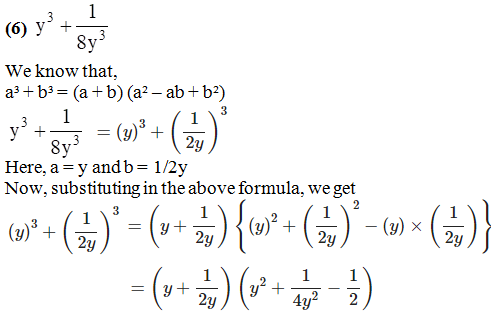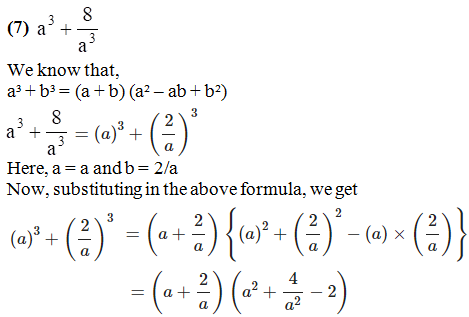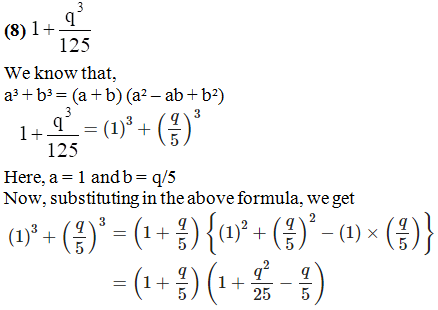Practice set 6.3 PAGE NO: 32

1. Factorize:

(1) y3 – 27

(2) x3 – 64y3

(3) 27m3 – 216n3

(4) 125y3 – 1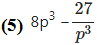(6) 343a3 – 512b3

(7) 64x2 – 729y2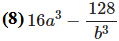Solution:

(1) y3 – 27

We know that,

a³ – b³ = (a – b) (a² + b² + ab)

y3 – 27 = y³ – (3)³

Here, a = y and b = 3

Now, substituting in the above formula, we get

y³ – (3)³ = (y – 3) [y² + y(3) + (3)2]

= (y – 3) (y² + 3y + 9)

(2) x3 – 64y3

We know that,

a³ – b³ = (a – b) (a² + b² + ab)

x3 – 64y3 = x³ – (4y)³

Here, a = x and b = 4y

Now, substituting in the above formula, we get

x³ – (4y)³ = (x – 4y) [x² + x(4y) + (4y)²]

= (x – 4y) (x² + 4xy + 16y²)

(3) 27m3 – 216n3

We know that,

a³ – b³ = (a – b) (a² + b² + ab)

27m3 – 216n3 = 27 (m³ – 8n³) [By taking out the common factor 27]

= 27 [m³ – (2n)³]

Here, a = m and b = 2n

Now, substituting in the above formula, we get

27 [m³ – (2n)³] = 27 {(m – 2n) [m² + m(2n) + (2n)²]}

= 27 (m – 2n) (m² + 2mn + 4n²)

(4) 125y3 – 1

We know that,

a³ – b³ = (a – b) (a² + b² + ab)

125y3 – 1= (5y)³ – 1³

Here, a = 5y and b = 1

Now, substituting in the above formula, we get

(5y)³ – 1³ = (5y – 1) [(5y)² + (5y)(1) + (1)²]

= (5y – 1) (25y² + 5y + 1)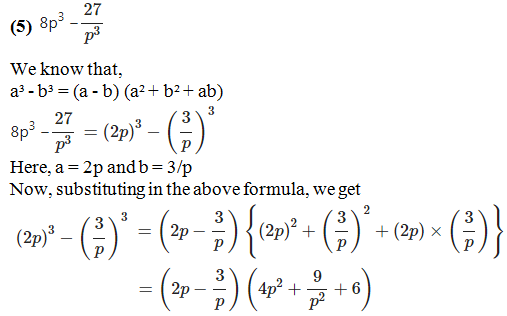(6) 343a3 – 512b3

We know that,

a³ – b³ = (a – b) (a² + b² + ab)

343a3 – 512b3 = (7a)³ – (8b)³

Here, a = 7a and b = 8b

Now, substituting in the above formula, we get

(7a)³ – (8b)³ = (7a – 8b) [(7a)² + (7a)(8b) + (8b)²]

= (7a – 8b) (49a² + 56ab + 64b²)

(7) 64x2 – 729y2

We know that,

a³ – b³ = (a – b) (a² + b² + ab)

64x2 – 729y2 = (4x)³ – (9y)³

Here, a = 4x and b = 9y

Now, substituting in the above formula, we get

(4x)³ – (9y)³ = (4x – 9y) [(4x)² + (4x) (9y) + (9y)²]

= (4x – 9y) (16x² + 36xy + 81y²)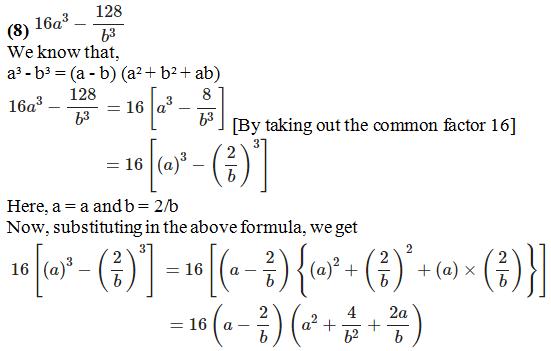2. Simplify:

(1) (x + y)3 – (x – y)3

(2) (3a + 5b)3 – (3a – 5b)3

(3) (a + b)3 – a3 – b3

(4) p3 – (p + 1)3

(5) (3xy – 2ab)3 – (3xy + 2ab)3

Solution:

(1) (x + y)3 – (x – y)3

Let us consider,

Here, a = x + y and b = x – y

By using the formula,

[a³ – b³ = (a – b) (a² + ab + b²)]

Let us simplify the given expression, we get

(x + y)3 – (x – y)3 = [(x + y) – (x – y)] [(x + y)² + (x + y) (x – y) + (x – y)]

= (x + y – x + y) [(x² + 2xy + y²) + (x² – y²) + (x² – 2xy + y²)]

= 2y(x² + x² + x² + 2xy – 2xy + y² – y² + y²)

= 2y (3x² + y²)

= 6x²y + 2y³

(2) (3a + 5b)3 – (3a – 5b)3

Let us consider,

Here, a = 3a + 5b and b = 3a – 5b

By using the formula,

[a³ – b³ = (a – b) (a² + ab + b²)]

Let us simplify the given expression, we get

(3a + 5b)3 – (3a – 5b)3 = [(3a + 5b) – (3a – 5b)] [(3a + 5b)² + (3a + 5b) (3a – 5b) + (3a – 5b)²]

= (3a + 5b – 3a + 5b) [(9a² + 30ab + 25b²) + (9a² – 25b²) + (9a² – 30ab + 25b²)]

= 10b (9a² + 9a² + 9a² + 30ab – 30ab + 25b² – 25b² + 25b²)

= 10b (27a² + 25b²)

= 270a²b + 250b³

(3) (a + b)3 – a3 – b3

By using the formula,

[a³ + b³ = a3 + b3 + 3a2b + 3ab2]

By substituting in the above equation, we get

(a + b)3 – a3 – b3 = a³ + 3a²b + 3ab² + b³ – a³ – b³

= 3a²b + 3ab²

(4) p3 – (p + 1)3

By using the formula,

[a³ + b³ = a3 + b3 + 3a2b + 3ab2]

By substituting in the above equation, we get

p3 – (p + 1)3 = p³ – (p³ + 3p² + 3p + 1)

= p³ – p³ – 3p² – 3p – 1

= – 3p² – 3p – 1

(5) (3xy – 2ab)3 – (3xy + 2ab)3

Let us consider,

Here, a = 3xy – 2ab and b = 3xy + 2ab

By using the formula,

[a³ – b³ = (a – b) (a² + ab + b²)]

Let us simplify the given expression, we get

(3xy – 2ab)3 – (3xy + 2ab)3 = [(3xy – 2ab) – (3xy + 2ab)] [(3xy – 2ab)² + (3xy – 2ab) (3xy + 2ab) + (3xy + 2ab)²]

= (3xy – 2ab – 3xy – 2ab) [(9x²y² – 12xyab + 4a²b²) + (9x²y² – 4a²b²) + (9x²y² + 12xyab + 4a²b²)]

= (- 4ab) (9x²y² + 9x²y² + 9x²y² – 12xyab + 12xyab + 4a²b² – 4a²b² + 4a²b²)

= (- 4ab) (27 xy² + 4a²b²)

= -108x²y²ab – 16a³b³

Practice set 6.4 PAGE NO: 33

1. Simplify: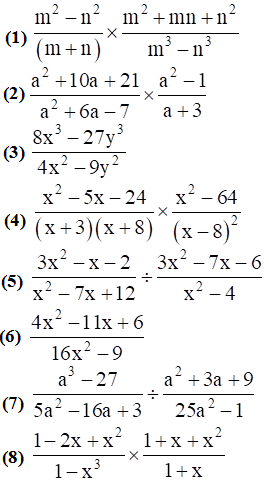Solution: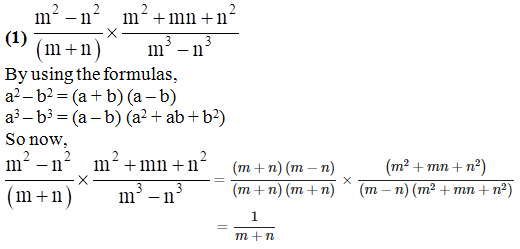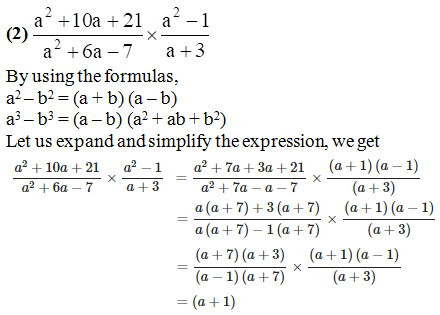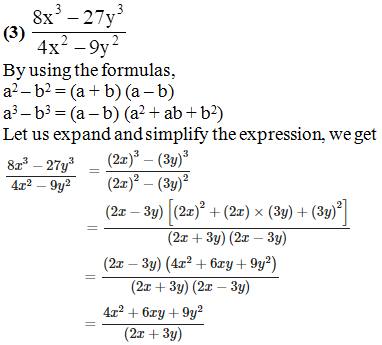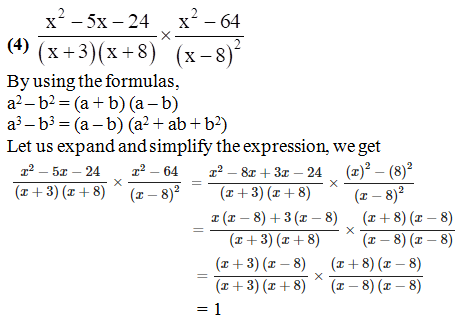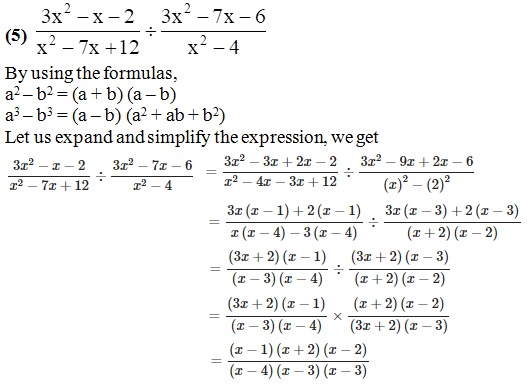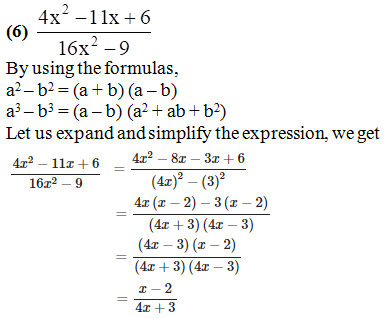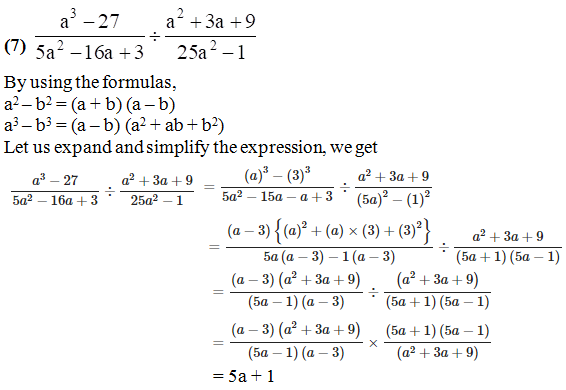Chapter 6 Factorisation of Algebraic Expressions provides useful resources for students as it helps them in scoring full marks in the examination.

Our experts have solved the difficult problems into simpler steps, which can be easily solved by students. Regular revision of important concepts and formulas over time is the best way to strengthen the concepts.

Many such exercise problems are given in the MSBSHSE Solutions book. Students can refer to them and can secure good marks in their examination.

## Frequently Asked Questions on Maharashtra State Board Solutions for Class 8 Maths Chapter 6 Factorisation of Algebraic Expressions

### Will these Maharashtra Board Class 8 Solutions be useful to prepare for the exams?

Yes, these solutions can be very helpful. Students are highly recommended to practise these solutions after revising the subjects, as they set the basis for the questions that could get asked often in the board exams.

### How are these Maharashtra State Board Class 8 Maths Chapter 6 Solutions helpful?

Students are encouraged to download these solutions and practise them. Then they can refer back to the solutions to analyse their performance. This will also help them to rectify their mistakes and to avoid making them during the board exams.

### Where can we find these Maharashtra State Board Class 8 Maths Chapter 6 Solutions?

Yes, we provide the solutions to download. We have also provided the solutions as a scrollable PDF and we have also mentioned the clickable link for the students to access. Meanwhile, the questions and the solutions  are also available online on our webpage.